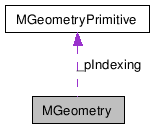# MGeometry Class Reference[OpenMayaRender - API module for rendering]

```#include <MGeometry.h>```
Collaboration diagram for MGeometry:[legend]

List of all members.

## Detailed Description

Geometric data cache.

MGeometry stores the collection of MGeometryData arrays which describe a Maya surface, including per-component data such as UV mapping and colour.

Examples:

## Public Member Functions

~MGeometry ()
MGeometry (const MGeometry &geom)
MGeometry operator= (const MGeometry &rhs)
unsigned int  primitiveArrayCount () const
const MGeometryPrimitive  primitiveArray (unsigned int arrayNumber) const
const MGeometryData  position ()
const MGeometryData  normal ()
const MGeometryData  componentId ()
const MGeometryData  texCoord (const MString &name)
const MGeometryData  color (const MString &name)
const MGeometryData  tangent (const MString &name)
const MGeometryData  binormal (const MString &name)
const MGeometryData  data (MGeometryData::DataType what, const MString &name)

## Friends

class  MGeometryManager
class  MGeometryList
class  MVaryingParameter

## Constructor & Destructor Documentation

 MGeometry::~MGeometry ( )

Class destructor.

 MGeometry::MGeometry ( const MGeometry & geom )

Copy constructor.

## Member Function Documentation

 MGeometry & MGeometry::operator= ( const MGeometry & rhs )

Copy constructor

Parameters:
 [in] rhs MGeometry to copy
Returns:
MGeometry copied into.

 unsigned int MGeometry::primitiveArrayCount ( ) const

Get the number of primitive data for this surface.

Returns:
Count is returned.
Examples:

 const MGeometryPrimitive MGeometry::primitiveArray ( unsigned int arrayNumber ) const

Get the primitive data for this surface. The primitive describes how the data arrays should be used when rendering.

Returns:
Primitive data structure which may be empty.
Examples:

 const MGeometryData MGeometry::position ( )

Get the position data for this surface.

Returns:
The position data (which may be empty).
Examples:

 const MGeometryData MGeometry::normal ( )

Get the normal data for this surface.

Returns:
The normal data (which may be empty).
Examples:

 const MGeometryData MGeometry::componentId ( )

Get the Maya component id data for this surface. This array allows you to correlate MGeometry entries with the corresponding component on the Maya surface (e.g. for meshes, this array will contains the vertex id).

Returns:
The component id data (which may be empty).

 const MGeometryData MGeometry::texCoord ( const MString & name )

Get a texture coordinate set by name.

Parameters:
 [in] name the name of the set to return
Returns:
The texture coordinate data (which may be empty).
Examples:

 const MGeometryData MGeometry::color ( const MString & name )

Get a color set by name.

Parameters:
 [in] name the name of the set to return
Returns:
The color data (which may be empty).
Examples:

 const MGeometryData MGeometry::tangent ( const MString & name )

Get a tangent set by name.

Parameters:
 [in] name the name of the uv set tangent data should be returned for
Returns:
The tangent data (which may be empty).
Examples:

 const MGeometryData MGeometry::binormal ( const MString & name )

Get a binormal set by name.

Parameters:
 [in] name the name of the uv set binormal data should be returned for
Returns:
The binormal data (which may be empty).
Examples:

 const MGeometryData MGeometry::data ( MGeometryData::DataType what, const MString & name )

Get arbitrary surface data by name.

Parameters:
 [in] what the type of data requested [in] name the name of the data set requested
Returns:
The geometry data (which may be empty).

 Autodesk® Maya® 2011 © 1997-2010 Autodesk, Inc. All rights reserved. Generated with1.5.6